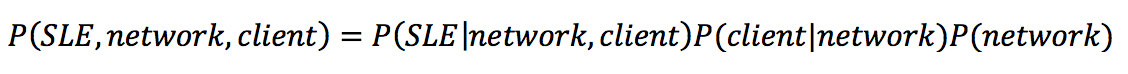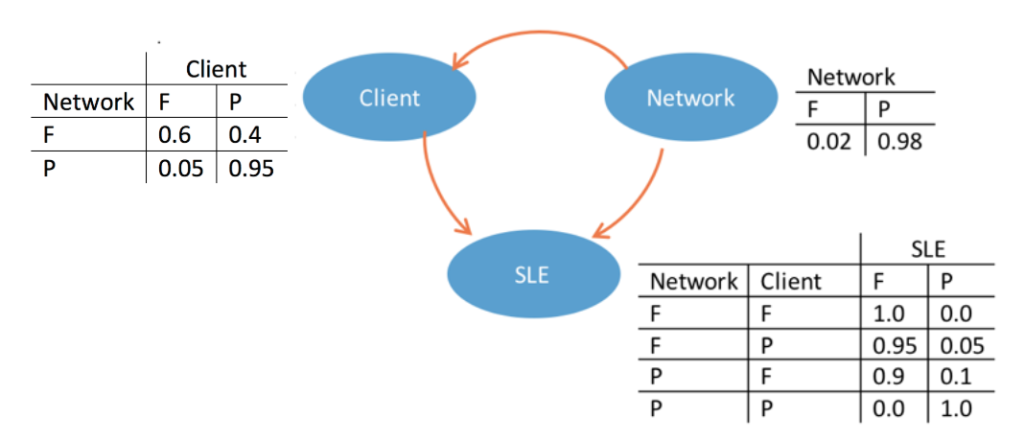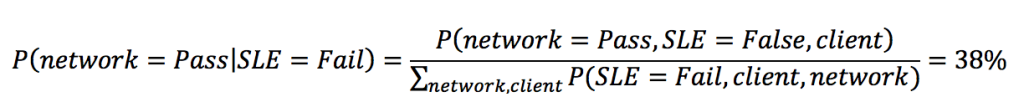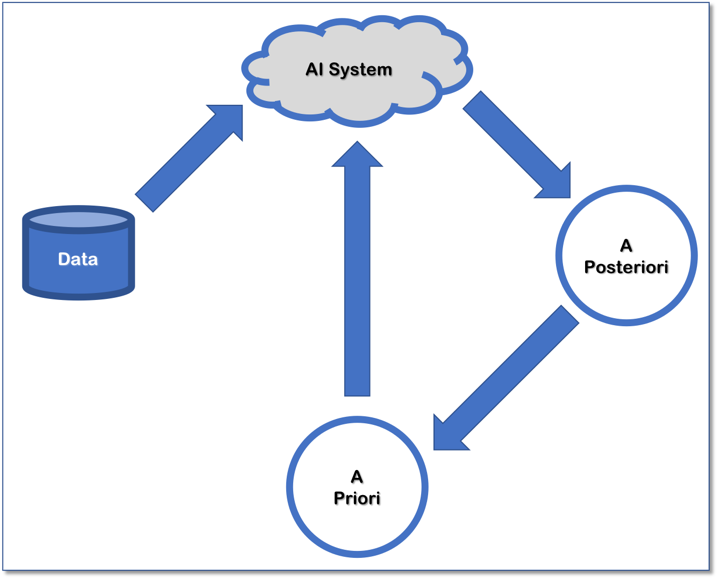# Bayesian Inference: a Key Building Block of an AI Foundation

9 Jul 2018 3:00am, byEbrahim Safavi
Ebrahim Safavi is data scientist at Mist Systems. He is an artificial intelligence enthusiast with experiences in areas ranging from information retrieval, knowledge discovery and natural language processing to the Internet of Things (IoT). He utilizes machine learning and data-driven analysis to solve real-world problems. Ebrahim has a Ph.D. degree on cognitive learning networks from Stevens Institute of Technology. He enjoys listening to music, hiking, and photography.

My first post discussed why mutual information, coupled with the Pearson correlation, is an important building block for AI technologies. Bayesian inference is another. Bayes’ theorem allows us to use some knowledge or belief that we already have, also known as the “prior,” to help us calculate the probability of a related event. This data mining method can be used in analyzing network variables in AI-powered network management systems, virtual assistants and other variable analytics models.

Bayesian inference is an extremely powerful set of tools for modeling any random variable, such as the coverage probability, location statistic, and Service Level Expectation (SLE) metrics, etc. Bayesian models map our understanding of a problem and evaluate observed data into a quantitative measure of how certain we are of a particular fact in probabilistic terms, where the probability of a proposition simply represents a degree of belief in the truth of that proposition.

Bayesians are uncertain about what is true; in a networking example, this means the coverage probability, location statistics and SLE metrics, etc. Bayesians use data as evidence that certain facts are more likely than others. Prior distributions reflect our beliefs before seeing any data, and posterior distributions reflect our beliefs after we have considered all the evidence.

For example, suppose that in a simple wireless network realization, there are two events that can affect an SLE metric — network feature and client feature — and all variables have two possible values, Fail or Pass.

Then we can use Bayes’ rule to find the joint probability function as:In Bayesian inference, our initial beliefs are represented by the prior distribution P(network), and our final beliefs are represented by posterior distributions P(client | network) and P(SLE | client, network).In the case of our simplified wireless network realization, client and network are hidden variables, and the only observable variable is the SLE metric. However, since the Bayesian network is a complete model for the variables and their dependencies, we can answer interventional questions about hidden variables like “what is the probability that network successfully passes given the SLE has failed (i.e., P(network=P | SLE=F))?”

We can use Bayes’ rule, the joint probability and conditional probability tables in the diagram to answer that question:The basic procedure of Bayesian methodology involves the following steps. First, assign an initial prior probability distribution, which quantifies all the relevant information into one distribution. Next, choose a probabilistic model that relates the random variables and the model parameters associated with the experiment. Lastly, apply Bayes theorem, to combine the prior knowledge and the new observed data to find the posterior probability distribution.

The posterior distribution is updated according to the data, meaning that the prior probability is changed by the new evidence provided by the data information, becoming posteriors. We can say that “Today’s posterior is tomorrow’s prior!There are several concepts which are beyond the scope of this tutorial but are very important for doing Bayesian analysis successfully, such as: how to choose a prior, the Bayesian network structure, or how to update prior beliefs based on data. Hopefully, this brief introduction to Bayesian methodology inspires you to continue exploring the fascinating world of Bayesian inference for AI systems.

Feature image via Pixabay.